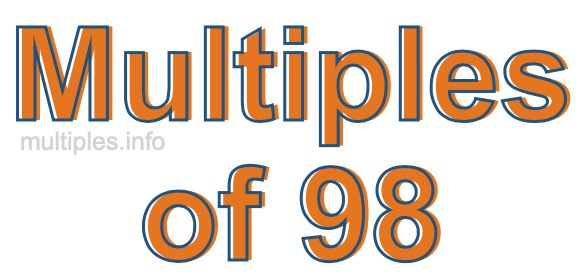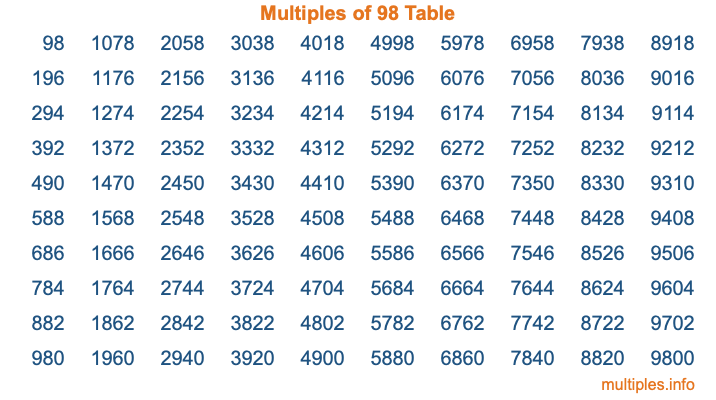Multiples of 98Welcome to the Multiples of 98 page. Here we will first teach you everything you will ever need to know about the multiples of 98, and then give you a study guide summary of everything we taught you to make sure you remember it all. Use this page to look up facts and learn information about the multiples of 98. This page will make you a multiples of ninety-eight expert!

Definition of Multiples of 98
Multiples of 98 are all the numbers that when divided by 98 equal an integer. Each of the multiples of 98 are called a multiple. A multiple of 98 is created by multiplying 98 by an integer.

Therefore, to create a list of multiples of 98, you start with 1 multiplied by 98, then 2 multiplied by 98, then 3 multiplied by 98, and so on for as long as you want. Thus, the list of the first five multiples of 98 is 98, 196, 294, 392, and 490. To see a larger list of multiples of 98, see the printable image of Multiples of 98 further down on this page. We also have a category where you can choose any nth multiple of 98.

Multiples of 98 Checker
The Multiples of 98 Checker below checks to see if any number of your choice is a multiple of 98. In other words, it checks to see if there is any number (integer) that when multiplied by 98 will equal your number. To do that, we divide your number by 98. If the the quotient is an integer, then your number is a multiple of 98.

Is  a multiple of 98?

Least Common Multiple of 98 and ...
A Least Common Multiple (LCM) is the lowest multiple that two or more numbers have in common. This is also called the smallest common multiple or lowest common multiple and is useful to know when you are adding our subtracting fractions. Enter one or more numbers below (98 is already entered) to find the LCM.

Check out our LCM Calculator if you need more details about the Least Common Multiple or if you need the LCM for different numbers for adding and subtraction fractions.

nth Multiple of 98
As we stated above, 98 is the first multiple of 98, 196 is the second multiple of 98, 294 is the third multiple of 98, and so on. Enter a number below to find the nth multiple of 98.

th multiple of 98

Multiples of 98 vs Factors of 98
98 is a multiple of 98 and a factor of 98, but that is where the similarities end. All postive multiples of 98 are 98 or greater than 98. All positive factors of 98 are 98 or less than 98.

Below is the beginning list of multiples of 98 and the factors of 98 so you can compare:

Multiples of 98: 98, 196, 294, 392, 490, etc.

Factors of 98: 1, 2, 7, 14, 49, 98

As you can see, the multiples of 98 are all the numbers that you can divide by 98 to get a whole number. The factors of 98, on the other hand, are all the whole numbers that you can multiply by another whole number to get 98.

It's also interesting to note that if a number (x) is a factor of 98, then 98 will also be a multiple of that number (x).

Multiples of 98 vs Divisors of 98
The divisors of 98 are all the integers that 98 can be divided by evenly. Below is a list of the divisors of 98.

Divisors of 98: 1, 2, 7, 14, 49, 98

The interesting thing to note here is that if you take any multiple of 98 and divide it by a divisor of 98, you will see that the quotient is an integer.

Multiples of 98 Table
Below is an image of the first 100 multiples of 98 in a table. The table is in chronological order, column by column. The first column has the first ten multiples of 98, the second column has the next ten multiples of 98, and so on.The Multiples of 98 Table is also referred to as the 98 Times Table or Times Table of 98. You are welcome to print out our table for your studies.

Negative Multiples of 98
Although not often discussed or needed in math, it is worth mentioning that you can make a list of negative multiples of 98 by multiplying 98 by -1, then by -2, then by -3, and so on, to get the following list of negative multiples of 98:

-98, -196, -294, -392, -490, etc.

Multiples of 98 Summary
Below is a summary of important Multiples of 98 facts that we have discussed on this page. To retain the knowledge on this page, we recommend that you read through the summary and explain to yourself or a study partner why they hold true.

There are an infinite number of multiples of 98.

A multiple of 98 divided by 98 will equal a whole number.

98 divided by a factor of 98 equals a divisor of 98.

The nth multiple of 98 is n times 98.

The largest factor of 98 is equal to the first positive multiple of 98.

98 is a multiple of every factor of 98.

98 is a multiple of 98.

A multiple of 98 divided by a divisor of 98 equals an integer.

98 divided by a divisor of 98 equals a factor of 98.

Any integer times 98 will equal a multiple of 98.

Multiples of a Number
Here you can get the multiples of another number, all with the same attention to detail as we did for multiples of 98 on this page.

Multiples of
Multiples of 99
Did you find our page about multiples of ninety-eight educational? Do you want more knowledge? Check out the multiples of the next number on our list!